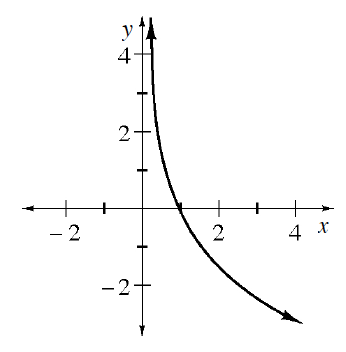### Home > INT3 > Chapter 6 > Lesson 6.3.1 > Problem6-82

6-82.

At right is a graph of $y = \log_b(x)$. Describe the possible values for $b$. Homework Help ✎

Think of the graph of $y = \log(x)$. How is this graph different?
How would that affect the equation?
What is the maximum possible value of $b$? What is the minimum?Use the eTool below to see how different values of $b$ change the graph.
Click the link at right for the full version of the eTool: INT3 6-82 HW eTool